# From Gen Chem to Org Chem Pt. 12 – Kinetics

Last updated: March 21st, 2021 |

Chemical kinetics is the study of reaction rates.  It can be really important in figuring out the mechanisms of chemical reactions. Here are the essentials of what you need to remember about chemical kinetics as you go from Gen chem to organic chemistry.

## 1. Reaction rates are proportional to concentration and to temperature.

First and foremost, chemical reactions occur when collisions occur between atoms or molecules and there is resulting change in the arrangement of the chemical bonds. Therefore,  rate of a reaction is proportional to the number of collisions between molecules.  Not every collision will result in a chemical reaction; of the total number of collisions, only a certain percentage will occur at the appropriate energy to allow the processes of chemical bond breaking and forming to occur. Think of a telemarketer selling Ginsu knives – only a small percentage of the calls he makes will result in a sale, but the total sales will be proportional to the number of calls.

In chemical reactions, anything which increases the collision rate will increase the reaction rate. One way to do this is to increase the concentration. Another is to increase the temperature -you might recall that what we call “temperature” can be looked at as a measure of atomic and molecular motion.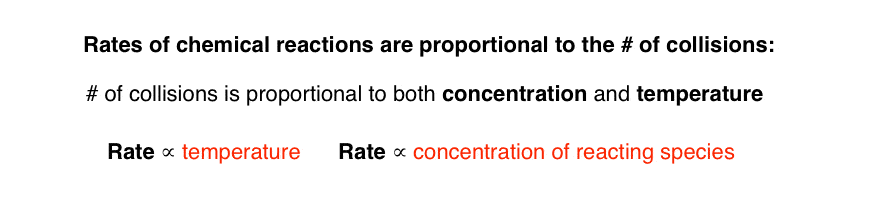## 2. First and Second order reactions

Depending on the type of reaction, either one or two chemical species can be involved in the transformation. Reactions involving more than two chemical species are almost never seen due to the improbability of three species all colliding at the same time with the right energy.

The order of a reaction is the number of species involved in the reaction.

The rate of a reaction is how many molecules of a compound are being made per unit volume per second, as expressed in M/L•s. We can write an equation for this if we take the proportionality relationship, rate [fish] conc, and change into an equation by introducing a constant k called the rate constant. Every reaction has a unique rate constant that is valid at constant temperature, which makes sense since increasing the temperature will increase the number of collisions and lead to a different rate.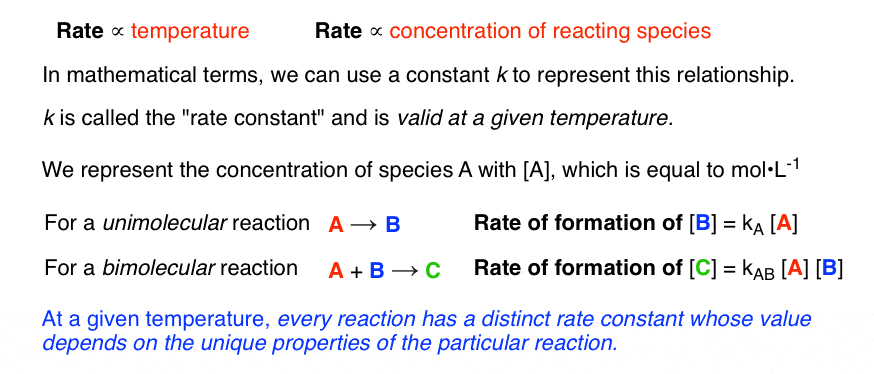For a unimolecular reaction involving just one species A,  the rate will be equal to  k[A] where [A] is the concentration of A in mol/L. For a bimolecular reaction  A+B, the rate will be equal to k[A][B] where [A] and [B] are the concentrations of A and B.

For instance in the SN1 reaction, which you’ll learn about in Org 1, the first step is the breaking of a weak bond to provide a halide (bromide in this example) and a carbocation. This is a first order, unimolecular reaction. We can write a rate constant for this reaction as rate = k [A]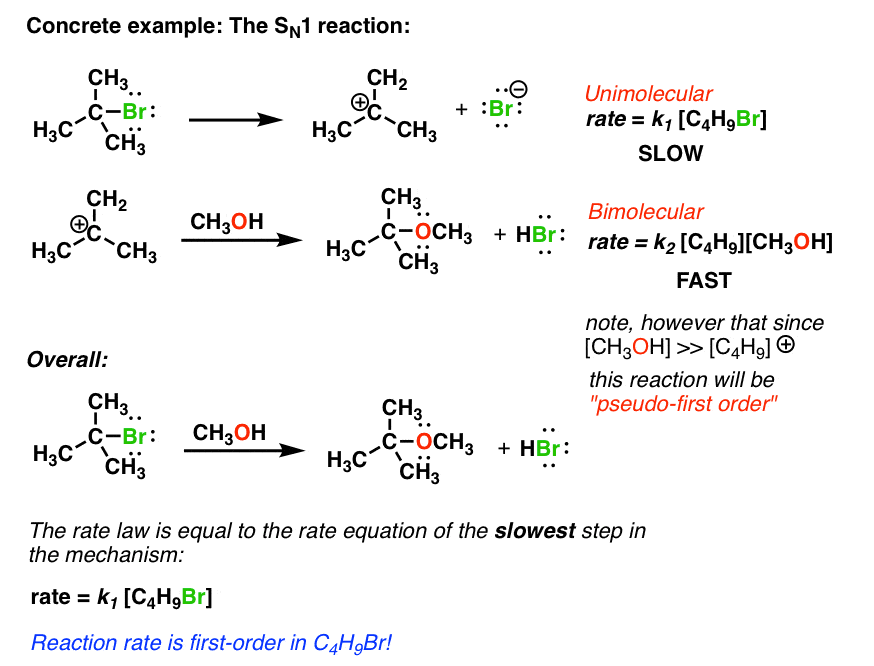The second step involves reaction of the carbocation with a molecule of solvent (methanol for example) to provide an ether. This is a second order reaction. Note here, however, that since the concentration of methanol is extremely high (it is the solvent) its concentration will be unaffected by the small amount of methanol consumed in this reaction. So the reaction rate will really only depend on the concentration of the carbocation, C4H9. This is an example of a pseudo-first order reaction.

Although there are many examples of reactions that involve just one overall transformation (like HCl + NaOH to give water, for instance), you’ll quickly discover in organic chemistry that reactions like the SN1 that proceed through more than one step are much more common. The sequence of reactions that lead to a particular product is referred to as the reaction mechanism.

## 3. The rate of a reaction is determined by the slowest step.

Here’s the thing about reaction rates: they’re determined by the rate of the slowest step. So in the example of the SN1 above, since first reaction is the slow step, we can approximate the rate of the reaction by the rate law for this step. So the overall rate law of the SN1 reaction, above, would be written  rate [C4H9OCH3] = k [C4H9Br]. In other words, the rate of formation of C4H9OCH3 at a given temperature is going to be directly proportional to the concentration of C4H9Br and won’t depend on the concentration of CH3OH at all.

## 4. Rate laws can give insight into the mechanism:

Here’s a useful tip:  we can actually use rate laws to give us information about mechanisms. For instance,  the SN1 reaction above is part of a general class of reactions called substitution reactions. There’s a second type of substitution mechanism called the SN2 reaction. The rate determining step of the SN1 is unimolecular, and the rate determining step of the SN2 is bimolecular.

Let’s change our example a bit from MeOH to the nucleophile I (–) (as a dissolved iodide salt like potassium iodide).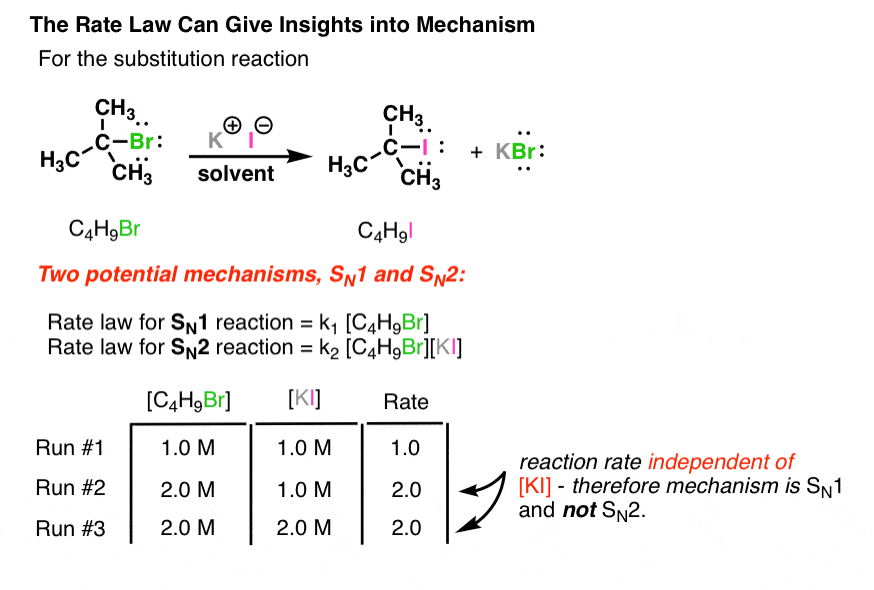Both reactions will give identical product C4H9I . How can we tell which mechanism operates?Simple! If the rate changes when we change the concentration of iodide [I(-)] we will know it is the SN2, and if it doesn’t change, it will be SN1.

In this example, the lack of change of the rate when we double the concentration of [I (-)] would imply that the mechanism is SN1 (or to be more precise, it would disprove the SN2, but that’s a whole other discussion).

## 5. The barrier to reaction is called the activation energy.

My second-year physical chemistry teacher made the concept of activation energy shockingly clear to us once by telling us that, in thermodynamic terms, the burning of human flesh to CO2 and water was extremely thermodynamically favorable, but our continued presence in his classroom was a testament to the existence of activation barriers. The point he was trying to make was that the thermodynamics of a process tells you nothing about the rate.

The rate of a reaction depends on a value called the activation energy, which is essentially the amount of energy required to reach a transition state that will lead to the formation of product.

The relationship between the activation energy, the temperature, and the reaction rate has been worked out in the form of the Arrhenius equation: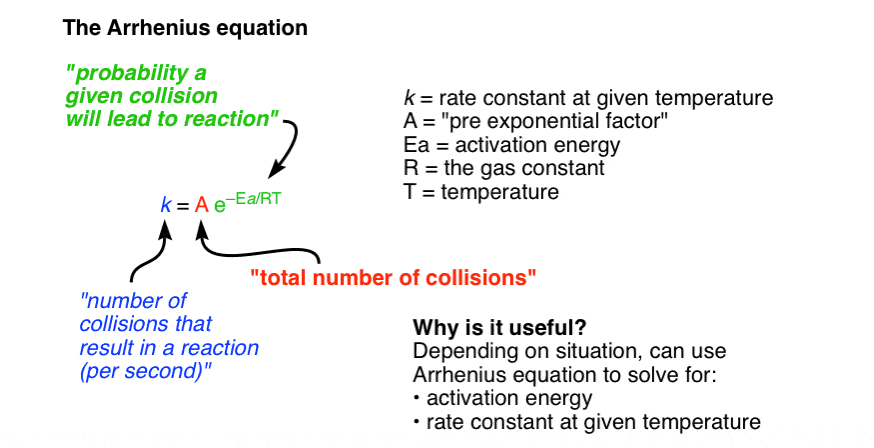In this equation k is the rate constant, T is the temperature, R is the gas constant, Ea is the activation energy, and the mysterious letter A is what’s known as the pre-exponential constant.

What it boils down to is that the rate constant k is the number of collisions that result in a reaction per second, A is the total # of collisions occuring per second, and e^(Ea/rt) is the probability that any given collision will result in a reaction.

Here’s a baseball analogy. Imagine you want to come up with an equation that will give you an estimate of the number of home runs hit in a given ballpark. You could come up with an equation that looked something like this:

# of home runs hit = [total # of balls hit ] [ probability ball has enough energy for a home run]

The “activation energy” in this case would be the distance from home plate to the outfield fences, which would be part of the “percentage” term (along with the height of the fence, presumably). As the fences get pushed back, the number of home runs will inevitably go down. If you wanted, you could find a way rearrange this equation so that you could figure out the distance to the fence if you knew the # of balls hit and the energy required to clear the fence.

The Arrhenius equation is very powerful and allows you to calculate the value of the activation energy as well as the value of the rate constant at different temperatures. In your course it’s doubtful you’ll be asked to go too deeply into the Arrhenius equation, but it’s still important to appreciate its subtleties.

Final note –  a useful rule of thumb from the Arrhenius equation is that the rate of the reaction doubles for every 10 degree incease in temperature.

Next Post – From Gen Chem to Organic Chem, Part 13 – Equilibria

## Comment section

### 8 thoughts on “From Gen Chem to Org Chem Pt. 12 – Kinetics”

1.Diana says:

Hey James! Thanks again for posting these quick and brief summaries of General Chemistry. I was reviewing some free-radical halogenation and I realized I forgot how to write a rate law. Thanks again for the refresher!

2.Sebastian says:

Hey James! Why do you use “the half of an infinity symbol” above. By the way, this is the best site ever. I’m learning Organic Chemistry before I take it in the Fall. Thank you

1.james says:

That means “proportional to”

3.Meredith says:

Thank you for this very useful website. I have stumbled onto it in preparation for my Org. Chem course that starts in a couple of weeks. This has been a really good review.

Question: I just want to clarify your final note… are you talking about the rate constant, or the rate itself?

Thanks.

4.Isabel says:

I love this tutorial,I like math, and I was very good at Chemical Reactores, but I never quite correlated the subject to practical examples. Thanks for doing so…

5.caters says:

I am confused about 3rd order and above reactions rarely occuring. In fact I disagree with this. Here is a good example of something that goes on and on and on from 2 of the simplest components, methane and chlorine:

The methane and chlorine are dissolved in each other so there is no solvent vs solute, just 2 reactants. The light comes(just plain sunlight can trigger this) and spontaneously the Cl2 breaks apart.

1) Cl2 + CH4 -> HCl + CH3Cl
2) sub 1) CH3Cl + CL2 -> CH2Cl2 + HCl
sub 2) 2 CH3Cl -> Cl2 + C2H6
3) sub 1) CH2Cl2 + Cl2 -> CHCl3 + HCl
sub 2) C2H6 + Cl2 -> C2H5Cl + HCl
etc.

This is of course free radical chlorination leading to every alkane and chlorocarbon(carbon with chlorine bonded to it) possible which is theoretically ∞.

Another example of this 3rd order and above would be if you took an alkyne, hydrogenated that to an alkene, then hydrogenated that to an alkane, than added a halogen to get an alkyl halide.

6.Mona says:

Hello James,
firstly, thank you for all your hard work. It is a great preparation for my upcoming org. chem. class.

In the image for SN1 Reaction C4H9Br -> Br- + C4H8
Where did the one Hydrogen atom go? Is this a mistake?
It would be great if you could clarify this as soon as possible!

1.James Ashenhurst says:

Error on the diagram. Fixed!

This site uses Akismet to reduce spam. Learn how your comment data is processed.